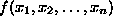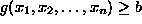Next: A prototype LP problem: Up: No Title Previous: No Title

# The LP formulation and the underlying assumptions

A Linear Programming problem is a special case of a Mathematical Programming problem. From an analytical perspective, a mathematical program tries to identify an extreme (i.e., minimum or maximum) point of a function, which furthermore satisfies a set of constraints, e.g.,. Linear programming is the specialization of mathematical programming to the case where both, function f - to be called the objective function - and the problem constraints are linear.

From an applications perspective, mathematical (and therefore, linear) programming is an optimization tool, which allows the rationalization of many managerial and/or technological decisions required by contemporary techno-socio-economic applications. An important factor for the applicability of the mathematical programming methodology in various application contexts, is the computational tractability of the resulting analytical models. Under the advent of modern computing technology, this tractability requirement translates to the existence of effective and efficient algorithmic procedures able to provide a systematic and fast solution to these models. For Linear Programming problems, the Simplex algorithm, discussed later in the text, provides a powerful computational tool, able to provide fast solutions to very large-scale applications, sometimes including hundreds of thousands of variables (i.e., decision factors). In fact, the Simplex algorithm was one of the first Mathematical Programming algorithms to be developed (George Dantzig, 1947), and its subsequent successful implementation in a series of applications significantly contributed to the acceptance of the broader field of Operations Research as a scientific approach to decision making.

As it happens, however, with every modeling effort, the effective application of Linear Programming requires good understanding of the underlying modeling assumptions, and a pertinent interpretation of the obtained analytical solutions. Therefore, in this section we discuss the details of the LP modeling and its underlying assumptions, by means of the following example.

UAL Data
Fri Jun 20 15:03:05 CDT 1997